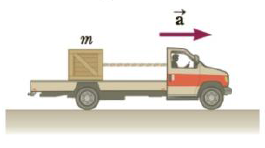Chapter 4, Problem 34P

Chapter
Section
Textbook Problem

A crate of mass m = 32 kg rides on the bed of a truck attached by a cord to the back of the cab as in Figure P4.34. The cord can with-stand a maximum tension of 68 N before breaking. Neglecting friction between the crate and truck bed, find the maximum acceleration the truck can have before the cord breaks.Figure P4.34

To determine
The maximum acceleration of the truck.

Explanation

Given info: The mass of the crate is 32 kg. The maximum tension on the cord is 68 N.

The free body diagram is given below.

From Newton’s second law, the formula for acceleration is,

amax=Tmaxm

• m is the mass of the crate
• Tmax is the maximum tension the cord can withstand

Substitute 32 kg for m and 68 N for Tmax in the above expression to get amax

Still sussing out bartleby?

Check out a sample textbook solution.

See a sample solution

The Solution to Your Study Problems

Bartleby provides explanations to thousands of textbook problems written by our experts, many with advanced degrees!

Get Started

As natural constituents of foods, phytochemicals are safe to consume in large amounts. T F

Nutrition: Concepts and Controversies - Standalone book (MindTap Course List)

(Chemical Connections 26D) What is a silent mutation?

Introduction to General, Organic and Biochemistry

How can you explain the Algol paradox?

Horizons: Exploring the Universe (MindTap Course List)